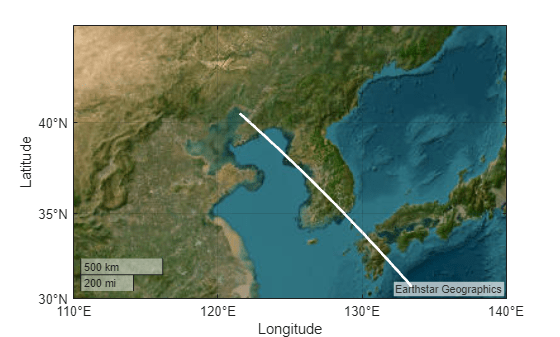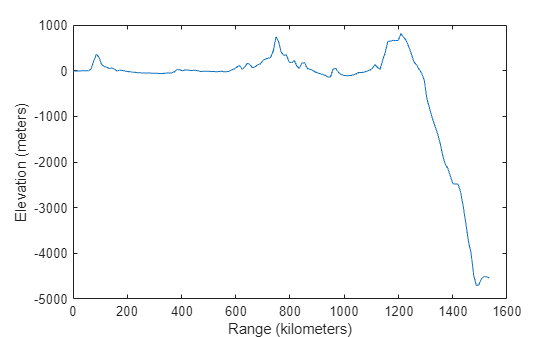Main Content

# Compute Elevation Profile Along Straight Line

Compute an elevation profile along a straight line using the `mapprofile` function. Calculating data values along a path is a common application when working with gridded geodata. For example, you might want to calculate the terrain height along a transect, a road, or a flight path. The `mapprofile` function does this, based on numerical data defining a set of waypoints, or by defining them interactively via graphic input from a map display. Values computed for the resulting profile can be displayed in a new plot or returned as output arguments for further analysis or display.

Load elevation data and a geographic cells reference object for the Korean peninsula.

`load korea5c`

Get the latitude and longitude limits of the elevation data and set up a world map. Display the map and apply a colormap appropriate for elevation data.

```latlim = korea5cR.LatitudeLimits; lonlim = korea5cR.LongitudeLimits; worldmap(latlim, lonlim) meshm(korea5c,korea5cR,size(korea5c),korea5c) demcmap(korea5c)```Define endpoints for a straight-line transect through the region. Then, compute the elevation profile using `mapprofile`. By default, `mapprofile` uses bilinear interpolation along a great circle track.

```plat = [40.5 30.7]; plon = [121.5 133.5]; [z,rng,lat,lon] = mapprofile(korea5c,korea5cR,plat,plon);```

Plot the transect in 3-D so it follows the terrain.

`plot3m(lat,lon,z,'w','LineWidth',2)`Plot the transect range and elevation on a set of Cartesian axes.

```figure plot(rng,z,'r')```## Support평가판 신청